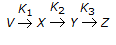# Chemical Engineering - Chemical Reaction Engineering

### Exercise :: Chemical Reaction Engineering - Section 1

21.

Which of the following is the most suitable for very high pressure gas phase reaction ?

 A. Batch reactor B. Tubular flow reactor C. Stirred tank reactor D. Fluidised bed reactor

Explanation:

No answer description available for this question. Let us discuss.

22.

The reaction between oxygen and organic material is a/an __________ reaction.

 A. exothermic B. endothermic C. biochemical D. photochemical

Explanation:

No answer description available for this question. Let us discuss.

23.

With decrease in temperature, the equilibrium conversion of a reversible endother-mic reaction

 A. decreases B. increases C. remains unaffected D. increases linearly with temperature

Explanation:

No answer description available for this question. Let us discuss.

24.

In an exothermic chemical reaction, the reactants compared to the products have

 A. higher temperature B. more energy C. less energy D. same energy.

Explanation:

No answer description available for this question. Let us discuss.

25.

For a reaction of the type,, the rate of reaction (- rx) is given by

 A. (K1+K1)CX B. (K1+K2+K3)CX C. K1CV - K2CX D. (K1-K2)CX